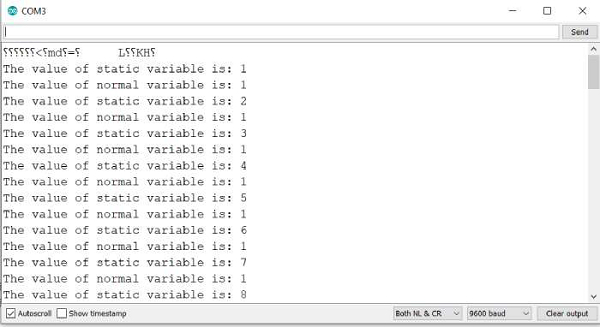# How to Use Static Variables in Arduino?

A static variable is a special kind of variable; it is allocated memory 'statically'. Its lifetime is the entire run of the program. It is specific to a function, i.e., only the function that defined it can access it. However, it doesn't get destroyed after the function call ends. It preserves its value between successive function calls. It is created and initialized the first time a function is called. In the next function call, it is not created again. It just exists.

## Example

Take a look at the following example.

void setup() {
Serial.begin(9600);
Serial.println();
}
void loop() {
staticFunctionDemo();
}
void staticFunctionDemo() {
static int staticVariable = 0;
int normalVariable = 0;
staticVariable = staticVariable+1;
normalVariable = normalVariable+1;
Serial.print("The value of static variable is: ");Serial.println(staticVariable);
Serial.print("The value of normal variable is: ");Serial.println(normalVariable);
}

## Output

The Serial Monitor output is shown below −As you can see, the normal variable is created and destroyed with each function call, whereas the static variable preserves its value in between function calls.